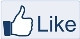# IMAGE PROCESSING

" Two roads diverged in a wood, and I,
I took the one less traveled by,
And that has made all the difference "-Robert Frost

### Lee filter using MATLAB

Lee filter for speckle reduction has been realized in MATLAB. For explanation check:
https://www.imageeprocessing.com/2014/08/lee-filter.html

MATLAB code:

%Add multiplicative noise to the image
J = imnoise(I,'speckle',0.01);
%Apply Lee filter
K = Lee_filter(I,J,[5 5]);
figure,subplot(121),imshow(J);title('Noisy Image');
subplot(122),imshow(uint8(K));title('Despeckled Image');

MATLAB Function:

function Y = Lee_filter(R,E,sz)
%R is the Reference Image
%E is the Error or Noisy Image
%K is the Kernel or Window
%Y is the Output Image

% Y = mean(K)+W*(C-mean(K);
% W = variance(K)/(variance(K)+variance(R))

%Define the type
R = double(R);
E = double(E);

%Preallocate the Output Matrix
Y = zeros(size(R));
mn = round((sz-1)/2);
Tot = sz(1,1)*sz(1,2);

%Variance of the reference Image
Rvar = var(R(:));

Indx = floor(median(1:Tot));
for i = 1:size(R,1)
for j = 1:size(R,2)
K = EImg(i:i+sz(1,1)-1,j:j+sz(1,2)-1);
varK = var(K(:));
meanK = mean(K(:));
W = varK./(varK+Rvar);

Y(i,j) = meanK + W * (K(Indx)-meanK);

end
end

end

EXPLANATION:

Save the function Lee-filter.m in separate fileLike "IMAGE PROCESSING" page